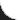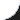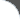Home | Tutorials | Articles | Videos | Products | Tools | Search
 Interviews | Open Source | Tag Cloud | Follow Us | Bookmark | Contact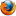In Browser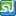StumbleUpon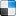del.icio.usGoogle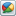Google Buzz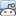redditLinkedInFacebook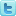TwitterLinkedinE-Mail
 Java > Core > Special Numbers > Prime Number

# Prime Number

Prime numbers are numbers that can only be divided by themselves or by one. i.e. A number is said to be prime if it is having one and itself as the only divisors. The following example shows how to check whether the given number is prime.

 File Name  : com/bethecoder/tutorials/core/special_numbers/PrimeNumber.java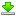Author  : Sudhakar KV Email  : [email protected]

 ``` package com.bethecoder.tutorials.core.special_numbers; /**  * A number is said to be prime if it is having one and itself as the only divisors.  *    * @author Sudhakar KV  *  */ public class PrimeNumber {   /**    * @param args    */   public static void main(String[] args) {     for (int i = 0 ; i < 20 ; i ++) {       if(isPrimeNumber(i)) {         System.out.println(i + " is a prime number");       } else {         System.out.println(i + " is not a prime number");       }     }   }   private static boolean isPrimeNumber(int number) {     boolean prime = true;     number = Math.abs(number);          if (number < 2) {       prime = false;     } else {       for (int i = 2 ; i <= number/2 ; i ++) {         if (number % i == 0) {           prime = false;           break;         }       }     }     return prime;   }    }```

It gives the following output,
```0 is not a prime number
1 is not a prime number
2 is a prime number
3 is a prime number
4 is not a prime number
5 is a prime number
6 is not a prime number
7 is a prime number
8 is not a prime number
9 is not a prime number
10 is not a prime number
11 is a prime number
12 is not a prime number
13 is a prime number
14 is not a prime number
15 is not a prime number
16 is not a prime number
17 is a prime number
18 is not a prime number
19 is a prime number
```